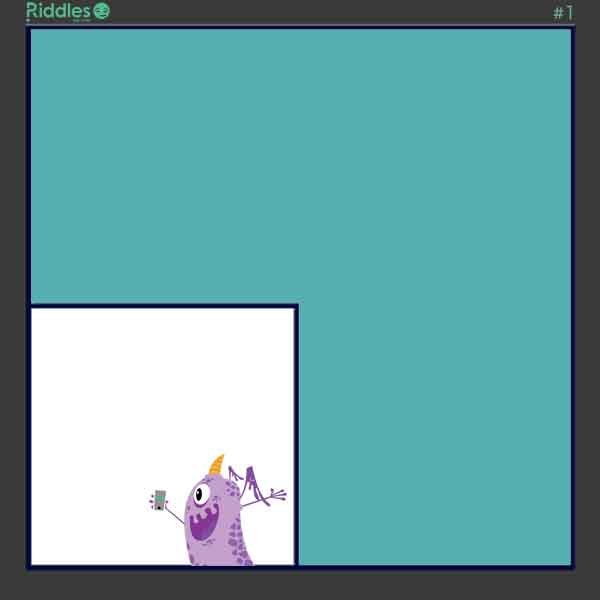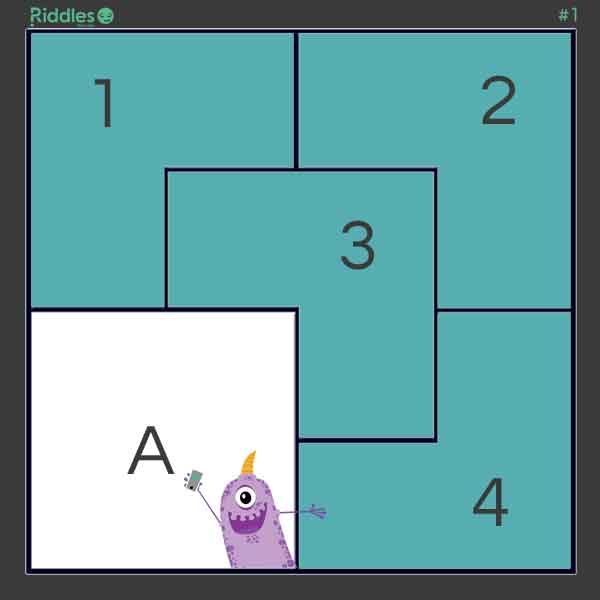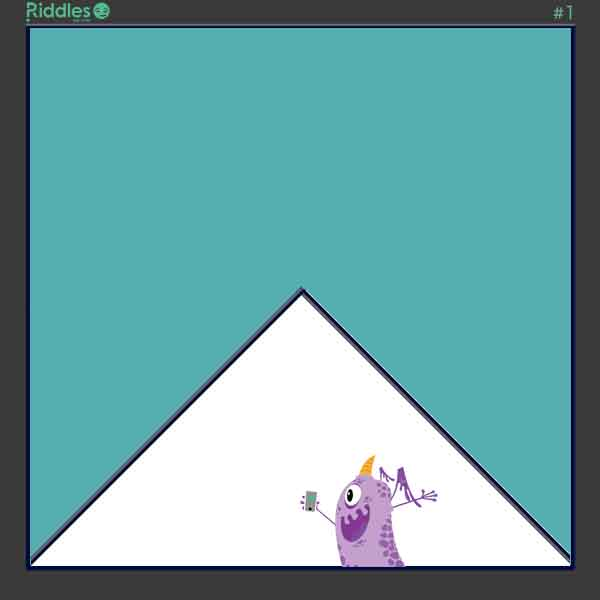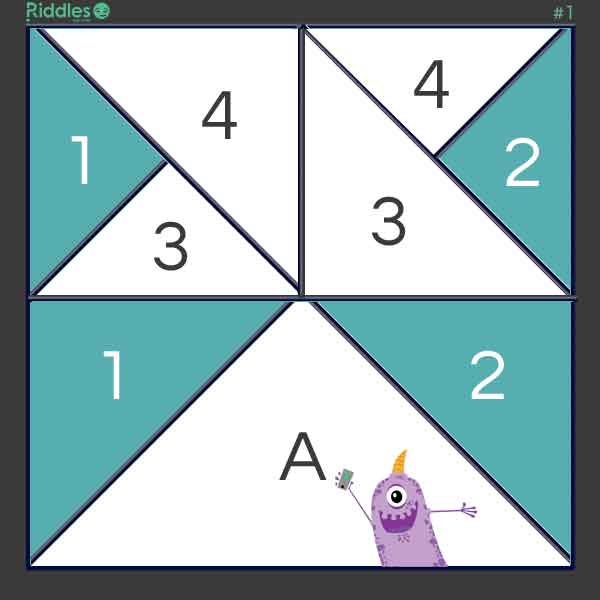Riddle: The word FACETIOUSLY contains the six vowels, A-E-I-O-U and Y, in their alphabetical order. Can you find another English word that does the same?
Answer: The word is abstemiously. There may be others.
Riddle: You have two container, a 5 gallon and a 3 gallon container. How do you measure out 4 gallons?
Answer: Fill up the 3 gallon container and pour the 3 gallons into the 5 gallon container.Then, fill the 3 gallon container back up, and pour it into the 5 gallon container.The 3 gallon container will have 1 gallon left. Empty the 5 gallon container.Pour the remining 1 gallon into the 5 gallon container.Then fill the 3 gallon container back up and pour it into the 5 gallon container.Thus, you have 4 gallons.
Riddle: It's always 1 to 6, it's always 15 to 20, it's always 5, but it's never 21, unless it's flying. What is it?
Answer: The answer is: a dice. An explanation: "It's always 1 to 6": the numbers on the faces of the dice, "it's always 15 to 20": the sum of the exposed faces when the dice comes to rest after being thrown, "it's always 5": the number of exposed faces when the dice is at rest, "but it's never 21": the sum of the exposed faces is never 21 when the dice is at rest, "unless it's flying": the sum of all exposed faces when the dice is flying is 21 (1 + 2 + 3 + 4 + 5 + 6).
Riddle: A boy leaves home in the morning to go to school. At the moment he leaves the house he looks at the clock in the mirror. The clock has no number indication and for this reason, the boy makes a mistake in interpreting the time (mirror-image). Just assuming the clock must be out of order, the boy cycles to school, where he arrives after twenty minutes. At that moment the clock at school shows a time that is two and a half hours later than the time that the boy saw on the clock at home. What time is it?
Answer: The difference between the real time and the time of the mirror image is two hours and ten minutes (two and a half hours, minus the twenty minutes of cycling). Therefore, the original time on the clock at home that morning could only have been five minutes past seven: The difference between these clocks is exactly 2 hours and ten minutes (note that also five minutes past one can be mirrored in a similar way, but this is not in the morning!). Conclusion: The boy reaches school at five minutes past seven plus twenty minutes of cycling, which is twenty-five minutes past seven!...
Riddle: How can this be true? Have a look at the picture. All the lines are straight, the shapes that make up the top picture are the same as the ones in the bottom picture so where does the gap come from?
Answer: The green triangle has dimensions 2 x 5 and gradient 2 / 5 = 0.4 The red triangle has dimensions 3 x 8 and gradient 3 / 8 = 0.375 Hence the gradient of the green triangle is greater than that of the red triangle.
Answer: Solution #1 - Squares First, Carl divides his as to reserve to himself one-fourth in the form of a square.Then, Carl takes the remaining 3/4 shape and scales it down by 1/4.  He then, multiplies the shape into 4 identically shaped pieces, and aranges them so that they fit into the original 3/4 shape.Solution #2 - Rectangles First, create a triangle that is 1/4 the size of the square.Now, with straight lines, create two squares. Proceed to disect the two squares with horizontal lines creating 4 triangles. Then, disect one of the resultuing triangles from each square.  The shape of land for each of his four children is divided evenly and is the same shape.